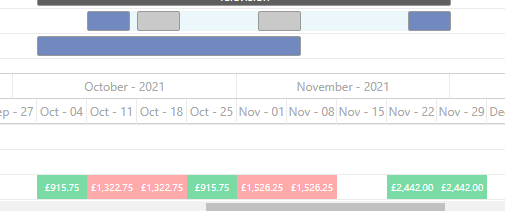# Percentage of task in scale cell

#1

Hi I’m trying to aggregate tasks in a resource view and I need to determine the percentage of a task within each scale cell to separate out a total dollar value.

Is there a way to get the start/end of a scale cell?#2

So for instance that longer blue bar spreads across 6 weeks, but the last week only has a fraction of the previous weeks. I would like to determine what percentage each vertical scale cell should be allocated, but I would need to determine at least the start/end of each scale cell - can that be retrieved? Is there an easier way to achieve this?

#3

Oof - I’m an idiot. The resource cell value gets passed the scales start and end… so I should be able to determine each tasks percentage from that.

``````gantt.templates.resource_cell_value = function(start_date, end_date, resource, tasks,
assignments){
return "<div>" + tasks.length * 8 + "</div>";
};``````